Stokes-Einstein relation

The Stokes-Einstein relation, originally derived by William Sutherland  but almost simultaneously published by Einstein , states that, for a sphere of radius$R$ immersed in a fluid,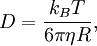$D=\frac{k_B T}{6\pi\eta R},$

where D is the diffusion constant,$k_B$ is the Boltzmann constant, T is the temperature and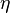$\eta$ is the viscosity. Sometimes, the name is given to the general relation: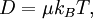$D=\mu k_B T,$

where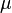$\mu$ is the mobility. This, coupled with Stokes' law for the drag upon a sphere moving though a fluid: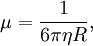$\mu=\frac{1}{6\pi\eta R} ,$

produces the first equation.